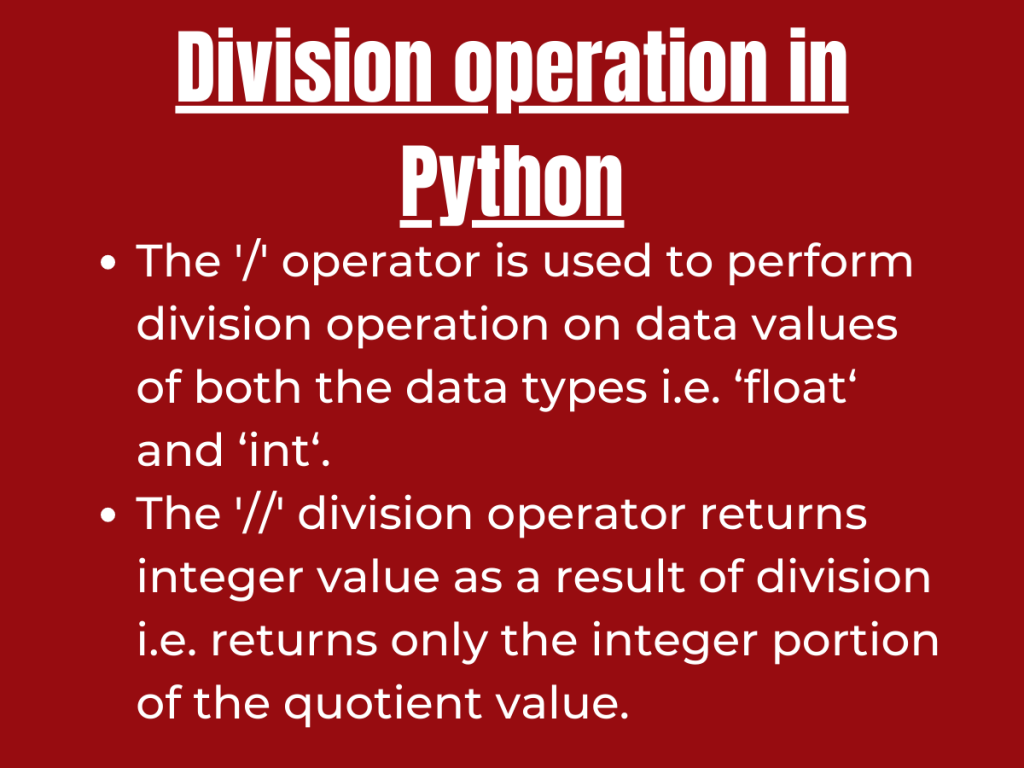# How to Perform the Python Division Operation?Hey, folks! In this article, we will be focusing on an arithmetic operation – Python Division operation.

## Getting started with Python division operation

Python has got various in-built operators and functions to perform arithmetic manipulations.

The `'/' operator` is used to perform division operation on data values of both the data types i.e. ‘float‘ and ‘int‘.

The beauty of Python ‘/’ operator is that this operator can handle decimal as well as negative values, respectively.

Syntax:

```number1 / number2
```

The operator operates on numeric values and returns a floating point value as a result. The result of the division operation is the Quotient of the operation performed, being represented as a floating point value.

Example 1:

```a = input("Enter the value for a:")
b = input("Enter the value of b:")
res = int(a)/int(b)
print(res)
```

Output:

```Enter the value for a:10
Enter the value of b:2
5.0
```

Example 2:

```a = -10
b = 20
res = a/b
print(res)
```

Output:

```-0.5
```

## Python division operation on Tuple

Python `floordiv() method` along with `map() function` can be used to perform division operation on various data values stored in a Tuple data structure.

Python `floordiv() method` is used to perform division operation on all the elements present in the data structure i.e. it performs element wise division operation. Further, `Python map() function` applies any passed/given function or operation on a set of iterables such as tuples, list, etc.

Syntax:

```tuple(map(floordiv, tuple1, tuple2))
```

The `floordiv() method` performs integer division i.e. divides the elements and returns only the integer portion of the quotient and skips the decimal portion.

Example:

```from operator import floordiv

inp_tup1 = (10,16,9,-4)
inp_tup2 = (2,-8,4,4)

tup_div = tuple(map(floordiv, inp_tup1, inp_tup2))

print("Resultant tuple after performing division operation : " + str(tup_div))

```

Output:

```Resultant tuple after performing division operation : (5, -2, 2, -1)
```

## Python division operation on Dict

Python division operation can be performed on the elements present in the dictionary using Counter() function along with ‘//’ operator.

The `Counter() function` stores the dictionary key-value data as dict keys and stores the count of the dict elements as the associated values.

The ‘//’ operator performs integer level division on the data elements.

Syntax:

```Counter({key : dict1[key] // dict2[key] for key in dict1})
```

Example:

```from collections import Counter

inp_dict1 = {'Python': 100, 'Java': 40, 'C': 36}
inp_dict2 = {'Python': 20, 'Java': -10, 'C': 8}

inp_dict1 = Counter(inp_dict1)
inp_dict2 = Counter(inp_dict2)
dict_div = Counter({key : inp_dict1[key] // inp_dict2[key] for key in inp_dict1})

print("Resultant dict after performing division operation : " + str(dict(dict_div)))
```

In the above example, we have stored the key-value pairs of the input dict in such a way using Counter() function that the input dict now contains the key as the dict elements and the value as the count of elements present in the dict.

Further, we have passed the keys to the ‘//’ operator to perform the division operation.

Output:

```Resultant dict after performing division operation : {'Python': 5, 'Java': -4, 'C': 4}
```

## Difference between Python ‘/’ and Python ‘//’ division operators

The basic and probably the only difference between the ‘/’ and ‘//’ division operators is that the` '/' operator` returns float values as the result of division i.e. it returns the entire quotient( the integer as well as the decimal part).

On the other hand,` '//' division operator` returns integer value as a result of division i.e. returns only the integer portion of the quotient value.

Example:

```print(10/3)
print(10//3)
```

Output:

```3.3333333333333335
3
```

## Conclusion

Thus, in this article, we have understood the ways to perform division operation in Python.

## References

• Python division operation